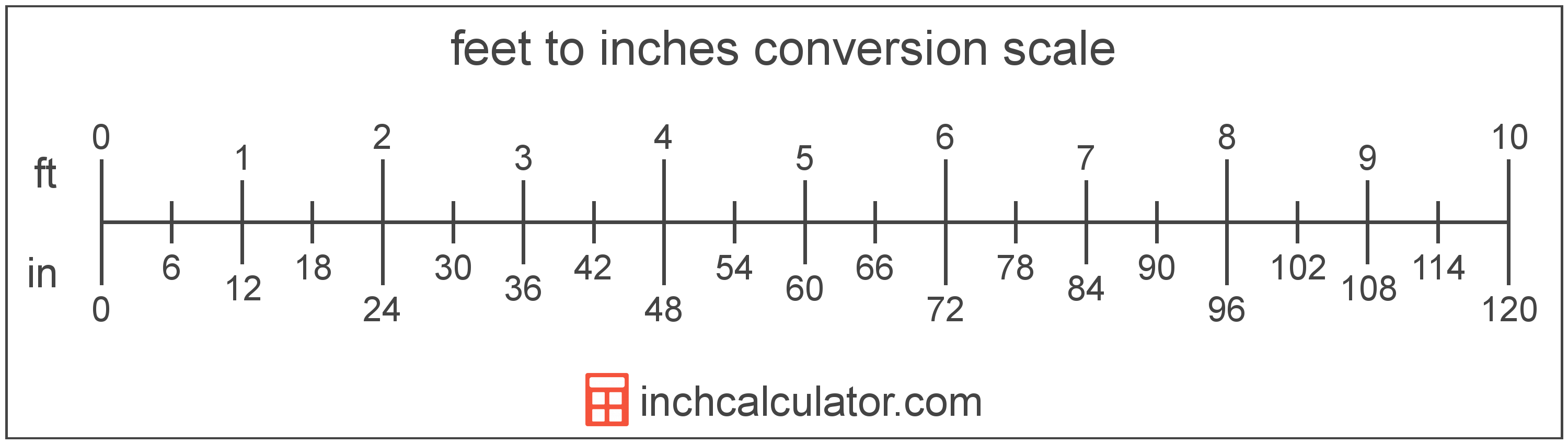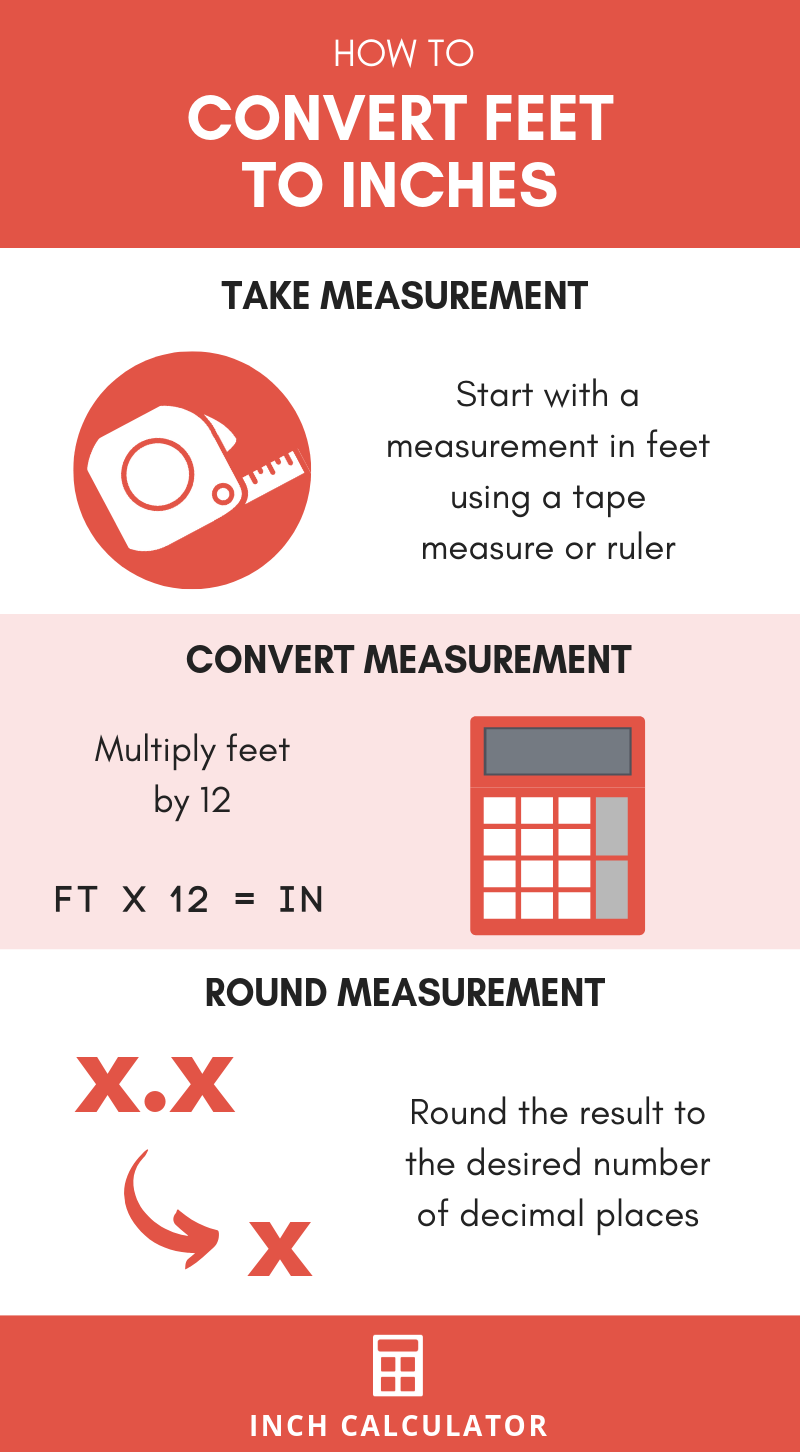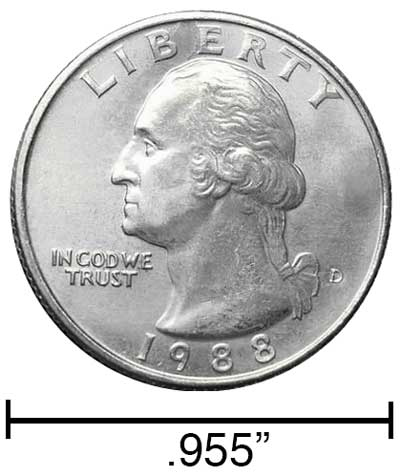# Feet to Inches Conversion Calculator

Enter the length in feet below to get the value converted to inches.

ft
in
Results in Inches:1 ft = 12 in
Do you want to convert inches to feet?

## How to Convert Feet to InchesTo convert a foot measurement to an inch measurement, multiply the length by the conversion ratio.

Since one foot is equal to 12 inches, you can use this simple formula to convert:

inches = feet × 12

The length in inches is equal to the feet multiplied by 12.

For example, here's how to convert 5 feet to inches using the formula above.
5' = (5 × 12) = 60"

### How Many Inches are in a Foot?

There are 12 inches in a foot, which is why we use this value in the formula above.

1' = 12"

Our inch fraction calculator can add feet and inches together and it also automatically converts the results to US customary, imperial and SI metric values.## Feet

The foot is a unit of linear length measure equal to 12 inches or 1/3 of a yard. Because the international yard is legally defined to be equal to exactly 0.9144 meters, one foot is equal to 0.3048 meters.

The foot is a US customary and imperial unit of length. Feet can be abbreviated as ft; for example, 1 foot can be written as 1 ft.

Feet can also be denoted using the symbol, otherwise known as a prime, though a single-quote (') is often used instead of the prime symbol for convenience. Using the prime symbol, 1 ft can be written as 1′.

The foot is most commonly measured using either a standard 12" ruler or a tape measure, though there are many other measuring devices available. Feet are sometimes referred to as linear feet, which are simply the measurement of length in feet.

You might be interested in our feet and inches calculator, which can add feet with inches, centimeters, or meters.

## Inches

An inch is a unit of linear length measure equal to 1/12 of a foot or 1/36 of a yard. Because the international yard is legally defined to be equal to exactly 0.9144 meters, one inch is equal to 2.54 centimeters.

The inch is a US customary and imperial unit of length. Inches can be abbreviated as in; for example, 1 inch can be written as 1 in.

Inches can also be denoted using the symbol, otherwise known as a double-prime. Often a double-quote (") is used instead of a double-prime for convenience. A double-prime is commonly used to express 1 in as 1″.The standard ruler has 12", and is a common measuring tool for measuring inches. They are also often measured using tape measures, which commonly come in lengths from 6' - 35'. Other types of measuring devices include scales, calipers, measuring wheels, micrometers, yardsticks, and even lasers.

We recommend using a ruler or tape measure for measuring length, which can be found at a local retailer or home center. Rulers are available in imperial, metric, or combination with both values, so make sure you get the correct type for your needs.

Need a ruler? Try our free downloadable and printable rulers, which include both imperial and metric measurements.

## Foot to Inch Conversion Table

Foot measurements converted to inches
Feet Inches
1' 12"
2' 24"
3' 36"
4' 48"
5' 60"
6' 72"
7' 84"
8' 96"
9' 108"
10' 120"
11' 132"
12' 144"
13' 156"
14' 168"
15' 180"
16' 192"
17' 204"
18' 216"
19' 228"
20' 240"

## References

1. National Institute of Standards and Technology, U.S. Survey Foot: Revised Unit Conversion Factors, https://www.nist.gov/pml/us-surveyfoot/revised-unit-conversion-factors
2. National Institute of Standards and Technology, Checking the Net Contents of Packaged Goods, Handbook 133 - 2019 Edition, https://nvlpubs.nist.gov/nistpubs/hb/2019/NIST.HB.133-2019.pdf